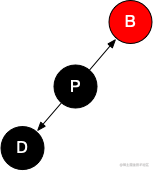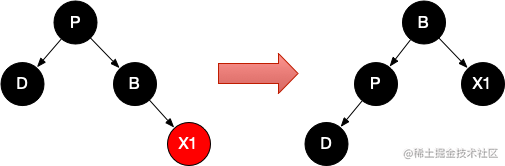# Java8 TreeMap之remove()方法

``````public V remove(Object key) {
Entry<K,V> p = getEntry(key);  // ①
if (p == null)
return null;

V oldValue = p.value;
deleteEntry(p); // ②
return oldValue;
}

①：找到要删除的节点

②：删除该节点

1. 没有子节点。无需替换，直接删除。
2. 有一个子节点。用子节点替换该节点即可。
3. 有2个子节点。这时就比较复杂了，节点删除后，这个空位由谁来顶替。打个比方，老头子死了，没立遗嘱，遗产由哪个子女继承？JDK8中使用后继节点的方法，来确定继承人。

``````if (p.left != null && p.right != null) {
Entry<K,V> s = successor(p); // ①
p.key = s.key; // ②
p.value = s.value;
p = s;
} // p has 2 children

①：p有两个子节点，符合情况3，获取p的后继节点。

②：执行替换

## 后继节点(successor)

### 后继节点的确认

• 如果节点有右子节点，则后继节点就是右子树中最小的节点。
• 如果节点没有右子节点，后继节点为某祖先节点，从当前节点往上找，如果它是父节点的右孩子，则继续找父节点，直到它不是右孩子或父节点为空，第一个非右孩子节点的父亲节点就是后继节点，如果父节点为空，则后继节点为null。
``````static <K,V> TreeMap.Entry<K,V> successor(Entry<K,V> t) {
if (t == null)
return null;
else if (t.right != null) { // 如果有右节点，获取右节点的最左节点，因为右节点大于父节点，左节点小于父节点
Entry<K,V> p = t.right;
while (p.left != null)
p = p.left;
return p;
} else { // 没有右子节点，查询祖先节点
Entry<K,V> p = t.parent;
Entry<K,V> ch = t;
while (p != null && ch == p.right) {
ch = p;
p = p.parent;
}
return p;
}
}

## 节点删除

### 情况一、有一个子节点

``````...

Entry<K,V> replacement = (p.left != null ? p.left : p.right);

if (replacement != null) {
replacement.parent = p.parent;
if (p.parent == null)
root = replacement;
else if (p == p.parent.left)
p.parent.left  = replacement;
else
p.parent.right = replacement;

// Null out links so they are OK to use by fixAfterDeletion.
p.left = p.right = p.parent = null;

...
}

...

### 情况二、无子节点

``````...

} else { //  No children. Use self as phantom replacement and unlink.
...

if (p.parent != null) {
if (p == p.parent.left)
p.parent.left = null;
else if (p == p.parent.right)
p.parent.right = null;
p.parent = null;
}
}

...

## 再平衡

1. 节点是红色或黑色。
2. 根是黑色。
3. 所有叶子都是黑色（叶子是NIL节点）。
4. 每个红色节点必须有两个黑色的子节点。（从每个叶子到根的所有路径上不能有两个连续的红色节点。）
5. 从任一节点到其每个叶子的所有简单路径都包含相同数目的黑色节点。

### 情况一、有一个子节点

#### 情况1.2、D为黑色

``````...
if (p.color == BLACK)
fixAfterDeletion(replacement);
...

``````private void fixAfterDeletion(Entry<K,V> x) {
while (x != root && colorOf(x) == BLACK) {
...
}
setColor(x, BLACK);
}

### 情况二、无子节点

#### 情况2.6、D为黑色，B为黑色，X1、X2不存在

1. D节点为红色，树是平衡状态。这时删除D节点，不影响树的平衡。
2. D节点为黑色，D节点所在的那条线多一个黑色节点，树不平衡。D被删除后，树自动平衡了。

### JDK8中实现

``````private void fixAfterDeletion(Entry<K,V> x) {
while (x != root && colorOf(x) == BLACK) {
if (x == leftOf(parentOf(x))) { // ① 如果x为左子节点
Entry<K,V> sib = rightOf(parentOf(x)); // ② x的兄弟节点

if (colorOf(sib) == RED) { // ③ 情况2.2，转换成情况2.6
setColor(sib, BLACK);
setColor(parentOf(x), RED);
rotateLeft(parentOf(x));
sib = rightOf(parentOf(x));
}

if (colorOf(leftOf(sib))  == BLACK &&
colorOf(rightOf(sib)) == BLACK) { // ④ 情况2.6
setColor(sib, RED);
x = parentOf(x);
} else {
if (colorOf(rightOf(sib)) == BLACK) { // ⑤ 情况2.4，转换成情况2.5
setColor(leftOf(sib), BLACK);
setColor(sib, RED);
rotateRight(sib);
sib = rightOf(parentOf(x));
}
// ⑥ 情况2.3、2.5，转换后的情况2.4
setColor(sib, colorOf(parentOf(x)));
setColor(parentOf(x), BLACK);
setColor(rightOf(sib), BLACK);
rotateLeft(parentOf(x));
x = root;
}
} else { // symmetric ⑦ 对称操作
Entry<K,V> sib = leftOf(parentOf(x));

if (colorOf(sib) == RED) {
setColor(sib, BLACK);
setColor(parentOf(x), RED);
rotateRight(parentOf(x));
sib = leftOf(parentOf(x));
}

if (colorOf(rightOf(sib)) == BLACK &&
colorOf(leftOf(sib)) == BLACK) {
setColor(sib, RED);
x = parentOf(x);
} else {
if (colorOf(leftOf(sib)) == BLACK) {
setColor(rightOf(sib), BLACK);
setColor(sib, RED);
rotateLeft(sib);
sib = leftOf(parentOf(x));
}
setColor(sib, colorOf(parentOf(x)));
setColor(parentOf(x), BLACK);
setColor(leftOf(sib), BLACK);
rotateRight(parentOf(x));
x = root;
}
}
}

setColor(x, BLACK);
}

①：先处理x作为左子节点的情况，这里的x相当于我们的D节点。

②：获取x的兄弟节点，这里的sib相当于我们的B节点。

③：情况2.2，转换成情况2.6

④：情况2.6，先将D的兄弟节点变成红色，然后让x指向P，这时P可黑可红，即x可能是黑色，也可能是红色。

``````  (1) 如果x为红色，不满足`colorOf(x) == BLACK`判断条件，退出循环。`setColor(x, BLACK);`，将x变成黑色，这时x所在的那条线比它的兄弟所在的那条线多一个黑色节点，满足上面说的结果2。由情况2.2转换成情况2.6时，x一定为红色。**情况2.2已处理完毕**

(2) 如果x为黑色，即情况2.6时P节点为黑色，P为红色时，上面已处理过了。

``````if (colorOf(sib) == RED) {
setColor(sib, BLACK);
setColor(parentOf(x), RED);
rotateLeft(parentOf(x));
sib = rightOf(parentOf(x));
}此时`sib`就是我们的P节点。 程序继续往下执行，`leftOf(sib)`就是我们的D节点，所以满足`colorOf(leftOf(sib)) == BLACK`条件。`rightOf(sib)`等于P的右节点，此时P的右节点为叶节点(NIL节点)，红黑树的规则3(所有叶子都是黑色)，所以满足`colorOf(rightOf(sib)) == BLACK`判断条件。进入代码块④，将P变为红色，之后和上面(1)中一样。情况2.6已处理完毕

⑤：情况2.4，转换成情况2.5

⑥：情况2.3、2.5，转换后的情况2.4。以情况2.5为例：之后`x = root`，退出循环。D被删除后，树又平衡了。

⑦：当x为右子节点时，和上面一样，做对称操作即可。这里不再赘述。